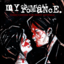MathematicsOpenStudy (anonymous):

Write an equation in slope-intercept form of the line that passes through (4, 8) and (0, -2).OpenStudy (amistre64):

(0,-2) IS your intercept so all you need to do is determine the slopeOpenStudy (amistre64):

subtract your points and stack y/x for the slopeOpenStudy (anonymous):

Could you guide me through the first step please?OpenStudy (amistre64):

(4 , 8) -(0,-2) ------ ? , ?OpenStudy (anonymous):

(4, 10) ?OpenStudy (amistre64):

good :) now slope = y/x; slope = 10/4, and reduceOpenStudy (anonymous):

Would y = 5/2 x - 2 be the answer?OpenStudy (amistre64):

now, since slope intercept forms is: y = slope x + intercept we just fill in the piecesOpenStudy (amistre64):

thats itOpenStudy (anonymous):

okay thanks :)OpenStudy (amistre64):

good jobOpenStudy (anonymous):

So for this would I do Determine the slope of the line which passes through these two points: (-1, 3) and (6, 9). (-1 , 3) - (6, 9) ----- ? ?

Latest Questionsshalong: What was John Calhounu2019s major objection to high tariffs?
25 minutes ago 0 Replies 0 Medalshayhay6: Give an example of an equation of a line with an undefined slope. What type of li
1 hour ago 1 Reply 0 Medalshayhay6: What is the equation of a line that passes through the point (5, -3) and has a sl
1 hour ago 1 Reply 0 Medalspiperkoebel: Does anyone know the coordinates of two points on the line y = u22122?
1 hour ago 1 Reply 0 Medalslolokrat: anyone good with microeconomics that can help me find out where i went wrong on m
12 hours ago 0 Replies 0 MedalsAsleepAndUnafraid21: URGENT PLZ HELP NOW Part C: Find the distance from B to E and from P to E. Show your work.
14 hours ago 49 Replies 5 MedalsKachirikutoka: How many solutions does the following equation have? |3x + 12| = 18 a No solution
18 hours ago 1 Reply 1 MedalAsleepAndUnafraid21: In the figure below, segment DE is parallel to segment BC and segment EF is parallel to AB: The figure shows triangle ABC with segments DE and DF.
15 hours ago 11 Replies 0 MedalsAsleepAndUnafraid21: In the figure below, segment DE is parallel to segment BC and segment EF is parallel to AB: The figure shows triangle ABC with segments DE and DF.
19 hours ago 1 Reply 0 Medalslakeawilliams: What is the solution to StartFraction 5 over 6 EndFraction x minus one-third grea
20 hours ago 1 Reply 0 Medals